## ML Aggarwal Class 7 Solutions for ICSE Maths Chapter 12 Congruence of Triangles Ex 12.1

Question 1.
If ΔABC and ΔDEF are congruent under the correspondence ABC ↔ FED, write all the corresponding congruent parts of the triangles.
Solution:
ΔABC and ΔDEF are congruent
under the correspondence, ABC ↔ FED∠A ↔ ∠F, ∠B ↔ ∠E, ∠C ↔ ∠D
AB ↔ FE, BC ↔ ED and AC ↔ FD

Question 2.
If ΔDEF = ΔBCA, then write the part(s) of ΔBCA that correspond to
(i) ∠E
(ii) $$\bar { EF }$$
(iii) ∠F
(iv) $$\bar { DF }$$
Solution:
If ΔDEF = ΔBCA, then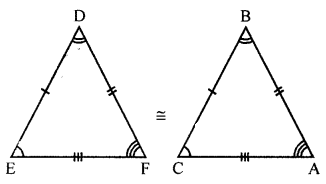(i) ∠E ↔ ∠C
(ii) EF ↔ $$\bar { CA }$$
(iii) ∠F ↔ ∠A
(iv) DF ↔ $$\bar { BA }$$

Question 3.
In the figure given below, the lengths of the sides of the triangles are indicated. By using SSS congruency rule, state which pairs of triangles are congruent. In the case of congruent triangles, write the result in symbolic form: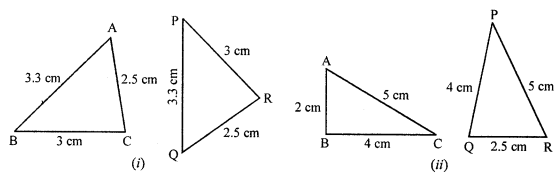Solution:
(i) In the given figure,
In ΔABC and ΔPQR
AB ↔ PQ, BC ↔ PR, and AC ↔ QR
Δs are congruent
ΔABC = ΔQPR
(ii) In the given figure,
In ΔABC and ΔPQR
AC ↔ PR, BC ↔ PQ
But AB ≠ QR
Δs are not congruent.

Question 4.
In the given figure, AB = 5 cm, AC = 5 cm, BD = 2.5 cm and CD = 2.5 cm
(i) State the three pairs of equal parts in ΔADB and ΔADC
(iii) Is ∠B = ∠C? Why?Solution:
In the given figure,
AB = 5 cm, AC = 5 cm, BD = 2.5 cm
and CD = 2.5 cm
In ΔABD and ΔACD
(i) AB = AC = 5 cm
BD = CD = 2.5 cm
ΔABD = ΔACD
∠B = ∠C (c.p.c.t.)Question 5.
In the given figure, AB = AC and D is the mid-point of $$\bar { BC }$$.
(i) State the three pairs of equal parts in ΔADB and ΔADC.
(iii) Is ∠B = ∠C? Why?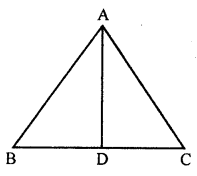Solution:
(i) In ΔABC,
AB = AC
D is the mid-point of BC
BD = DC
AB = AC (Given)
BD = DC (D is mid-point of BC)
(iii) ∠B = ∠C (c.p.c.t.)

Question 6.
In the figure given below, the measures of some parts of the triangles are indicated. By using SAS rule of congruency, state which pairs of triangles are congruent. In the case of congruent triangles, write the result in symbolic form.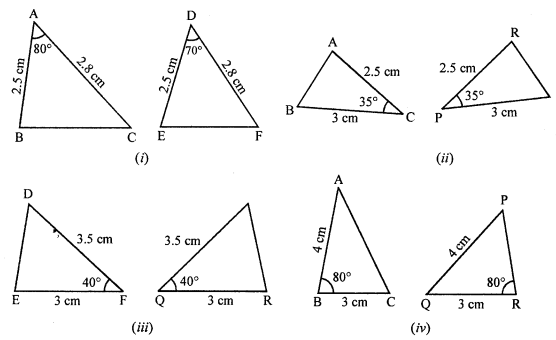Solution:
(i) In ΔABC and ΔDEF
AB = DE (Each = 2.5 cm)
AC = DF (Each = 2.8 cm)
∠A ≠ ∠D (Have different measure)
ΔABC is not congruent to ΔDEF
(ii) In ΔABC and ΔRPQ
AC = RP (Each = 2.5 cm)
CB = PQ (Each = 3 cm)
∠C = ∠P (Each = 35°)
ΔACB and ΔRPQ are congruent (SAS axiom)
(iii) In ΔDEF and ΔPQR
FD = QP (Each = 3.5 cm)
FE = QR (Each = 3 cm)
∠F = ∠Q (Each 40°)
(iv) In ΔABC and ΔPRQ
AB = PQ (Each = 4 cm)
BC = QR (Each = 3 cm)
But included angles B and ∠Q are not equal
ΔABC and ΔPQR are not congruent.

Question 7.
By applying SAS congruence rule, you want to establish that ΔPQR = ΔFED. If is given that PQ = EF and RP = DF. What additional information is needed to establish the congruence?
Solution:
In ΔPQR and ΔFED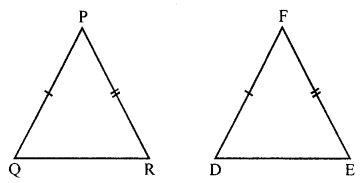PQ = FE
RP = DF
Their included angles ∠P must be equal to ∠F for congruency.
Hence, ∠P = ∠F

Question 8.
You want to show that ΔART = ΔPEN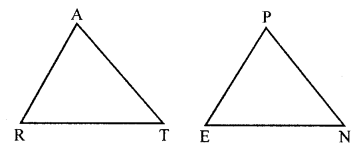(a) If you have to use SSS criterion, then you need to show
(i) AR = ……….
(ii) RT = ……….
(iii) AT = …………
(b) If it is given that ∠T = ∠N and you are to use the SAS criterion, you need to have
(i) RT = ………. and (ii) PN = ……….
Solution:
(a) In ΔART and ΔPEN
For SSS criterion
AR = DE
RT = EN and AT = PN
ΔART = ΔPEN
(b) ∠T = ∠N (Given)
In ΔART and ΔPEN
If RT = EN
AT = PN and ∠T = ∠N
Then ΔART = ΔPEN (SAS criterion)

Question 9.
You have to show that ΔAMP = ΔAMQ.
In the following proof, supply the missing reasons.

 Steps Reasons (i) PM = QM (i) ……… (ii) ∠PMA = ∠QMA (ii) ………. (iii) AM = AM (iii) ………… (iv) ΔAMP = ΔAMQ (iv) …………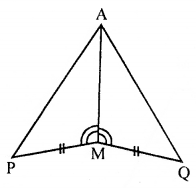Solution:
In order to show that,
ΔAMP = ΔAMQ
PM = QM (Given)
∠PMA = ∠QMA (Given)
AM = AM (Common)
ΔAMP = ΔAMQ (SAS criterion)

Question 10.
In the given figure:
(i) State three pairs of equal parts in ΔPSR and ΔRQP.
(ii) Is ΔPSR = ΔRQP? Give reasons
(iii) Is PS = RQ? Why?
(iv) Is ∠S = ∠Q? Why?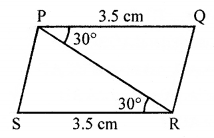Solution:
In ΔPSR and ΔRQP
SR = PQ (each = 3.5 cm)
PR = PR (Common side)
∠SRP = ∠RPQ (Each = 30°)
ΔPSR = ΔRQP (SAS criterion)
PS = RQ (c.p.c.t.)
∠S = ∠Q (c.p.c.t.)

Question 11.
In the given figure, AB = DC and ∠ABC = ∠DCB.
(i) State three pairs of equal parts in AABC and ADCB.
(ii) Is ΔABC = ΔDCB? Give reasons.
(iii) Is AC = DB? Why?Solution:
In ΔABC and ΔDBC
AB = DC (Given)
∠ABC = ∠DCB (Given)
BC = BC (Common)
ΔABC = ΔDCB (SAS criterion)
AC = DB (c.p.c.t.)

Question 12.
(i) State three pairs of equal parts in ΔABC and ΔABD.
(ii) Is ΔABC = ΔABD? Give reasons.
(iii) Is BC = BD? Why?
(iv) Is ∠C = ∠D? Why?Solution: